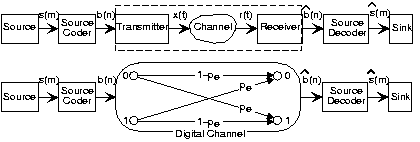# 6.19: Digital Channels

Learning Objectives

• An introduction to the components and structure of a digital channel.

Let's review how digital communication systems work within the Fundamental Model of Communication. As shown in Fig. 6.19.1, the message is a single bit. The entire analog transmission/reception system, which is discussed in various sections in this book can be lumped into a single system known as the digital channel.Fig. 6.19.1 The steps in transmitting digital information are shown in the upper system, the Fundamental Model of Communication. The symbolic-valued signal forms the message, and it is encoded into a bit sequence The indices differ because more than one bit/symbol is usually required to represent the message by a bitstream. Each bit is represented by an analog signal, transmitted through the (unfriendly) channel, and received by a matched-filter receiver. From the received bitstream the received symbolic-valued signal is derived.The lower block diagram shows an equivalent system wherein the analog portions are combined and modeled by a transition diagram, which shows how each transmitted bit could be received. For example, transmitting a 0 results in the reception of a 1 with probability pe (an error) or a 0 with probability 1 - pe (no error).

Digital channels are described by transition diagrams, which indicate the output alphabet symbols that result for each possible transmitted symbol and the probabilities of the various reception possibilities. The probabilities on transitions coming from the same symbol must sum to one. For the matched-filter receiver and the signal sets we have seen, the depicted transition diagram, known as a binary symmetric channel, captures how transmitted bits are received. The probability of error pe is the sole parameter of the digital channel, and it encapsulates signal set choice, channel properties, and the matched-filter receiver. With this simple but entirely accurate model, we can concentrate on how bits are received.

## Contributor

• ContribEEOpenStax